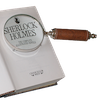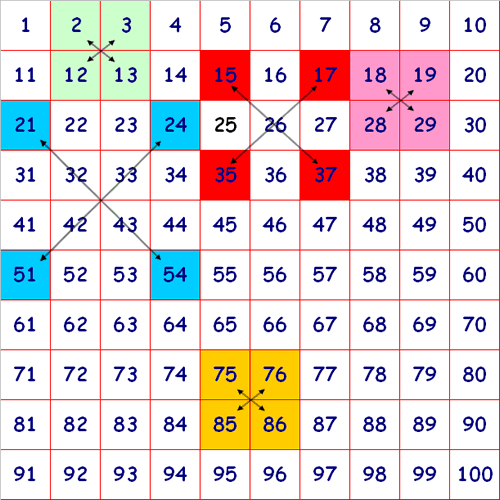You may also likeDouble DigitNumber Detective

Follow the clues to find the mystery number.Six Is the Sum

What do the digits in the number fifteen add up to? How many other numbers have digits with the same total but no zeros?

Diagonal Sums

Age 7 to 14Challenge Level

Here is a $100$ square with some of the numbers shaded:Look at the green square which contains the numbers $2, 3, 12$ and $13$.
Do you notice anything about the sum of the numbers that are diagonally opposite each other?

Look at the pink square.
What happens this time when you look at the numbers diagonally opposite each other?
Look at the squares shaded red. They form the corners of a large $3$ by $3$ square.